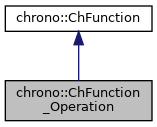chrono::ChFunction_Operation Class Reference

Description

Operation between functions:

math operation between A and B operands

• fa = first operand function
• fb = second operand function

#include <ChFunction_Operation.h>

Inheritance diagram for chrono::ChFunction_Operation:[legend]
Collaboration diagram for chrono::ChFunction_Operation:[legend]

Public Types

enum  eChOperation {
ChOP_ADD = 0, ChOP_SUB, ChOP_MUL, ChOP_DIV,
ChOP_POW, ChOP_MAX, ChOP_MIN, ChOP_MODULO,
ChOP_FABS, ChOP_FUNCT
}
Type of operation.Public Types inherited from chrono::ChFunction
enum  FunctionType {
FUNCT_CUSTOM, FUNCT_CONST, FUNCT_CONSTACC, FUNCT_DERIVE,
FUNCT_FILLET3, FUNCT_INTEGRATE, FUNCT_MATLAB, FUNCT_MIRROR,
FUNCT_MOCAP, FUNCT_NOISE, FUNCT_OPERATION, FUNCT_OSCILLOSCOPE,
FUNCT_POLY, FUNCT_POLY345, FUNCT_RAMP, FUNCT_RECORDER,
FUNCT_REPEAT, FUNCT_SEQUENCE, FUNCT_SIGMA, FUNCT_SINE,
FUNCT_LAMBDA
}
Enumeration of function types.

Public Member Functions

ChFunction_Operation (const ChFunction_Operation &other)

virtual ChFunction_OperationClone () const override
"Virtual" copy constructor (covariant return type).

virtual FunctionType Get_Type () const override
Return the unique function type identifier.

virtual double Get_y (double x) const override
Return the y value of the function, at position x.

void Set_optype (eChOperation m_op)

eChOperation Get_optype ()

void Set_fa (std::shared_ptr< ChFunction > m_fa)

std::shared_ptr< ChFunctionGet_fa ()

void Set_fb (std::shared_ptr< ChFunction > m_fb)

std::shared_ptr< ChFunctionGet_fb ()

virtual void Estimate_x_range (double &xmin, double &xmax) const override
Return an estimate of the range of the function argument. More...

virtual void ArchiveOUT (ChArchiveOut &marchive) override
Method to allow serialization of transient data to archives.

virtual void ArchiveIN (ChArchiveIn &marchive) override
Method to allow de-serialization of transient data from archives.Public Member Functions inherited from chrono::ChFunction
ChFunction (const ChFunction &other)

virtual double Get_y_dx (double x) const
Return the dy/dx derivative of the function, at position x. More...

virtual double Get_y_dxdx (double x) const
Return the ddy/dxdx double derivative of the function, at position x. More...

virtual double Get_weight (double x) const
Return the weight of the function (useful for applications where you need to mix different weighted ChFunctions)

virtual void Estimate_y_range (double xmin, double xmax, double &ymin, double &ymax, int derivate) const
Return an estimate of the range of the function value. More...

virtual double Get_y_dN (double x, int derivate) const
Return the function derivative of specified order at the given point. More...

virtual void Update (const double x)
Update could be implemented by children classes, ex. to launch callbacks.

virtual double Compute_max (double xmin, double xmax, double sampling_step, int derivate) const
Compute the maximum of y(x) in a range xmin-xmax, using a sampling method.

virtual double Compute_min (double xmin, double xmax, double sampling_step, int derivate) const
Compute the minimum of y(x) in a range xmin-xmax, using a sampling method.

virtual double Compute_mean (double xmin, double xmax, double sampling_step, int derivate) const
Compute the mean value of y(x) in a range xmin-xmax, using a sampling method.

virtual double Compute_sqrmean (double xmin, double xmax, double sampling_step, int derivate) const
Compute the square mean val. of y(x) in a range xmin-xmax, using sampling.

virtual double Compute_int (double xmin, double xmax, double sampling_step, int derivate) const
Compute the integral of y(x) in a range xmin-xmax, using a sampling method.

virtual double Get_Ca_pos () const
Computes the positive acceleration coefficient (inherited classes should customize this).

virtual double Get_Ca_neg () const
Compute the positive acceleration coefficient (inherited classes should customize this).

virtual double Get_Cv () const
Compute the speed coefficient (inherited classes must customize this).

virtual int HandleNumber () const
Return the number of handles of the function.

virtual bool HandleAccess (int handle_id, double mx, double my, bool set_mode)
Get the x and y position of handle, given identifier. More...

virtual int FilePostscriptPlot (ChFile_ps *m_file, int plotY, int plotDY, int plotDDY)
Plot function in graph space of the ChFile_ps postscript file where zoom factor, centering, color, thickness etc. More...

virtual int FileAsciiPairsSave (ChStreamOutAscii &m_file, double xmin=0, double xmax=1, int msamples=200)
Save function as X-Y pairs separated by space, with CR at each pair, into an ASCII file. More...

◆ Estimate_x_range()

 void chrono::ChFunction_Operation::Estimate_x_range ( double & xmin, double & xmax ) const
overridevirtual

Return an estimate of the range of the function argument.

(Can be used for automatic zooming in a GUI)

Reimplemented from chrono::ChFunction.

The documentation for this class was generated from the following files:
• /builds/uwsbel/chrono/src/chrono/motion_functions/ChFunction_Operation.h
• /builds/uwsbel/chrono/src/chrono/motion_functions/ChFunction_Operation.cpp